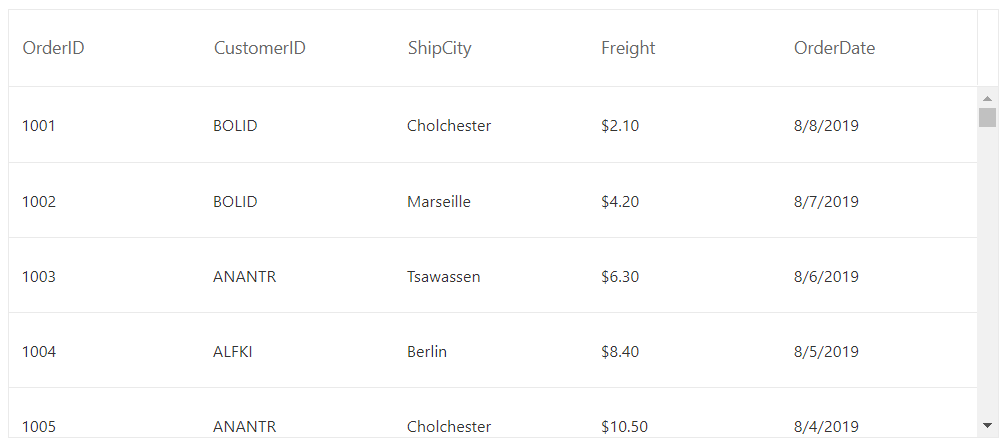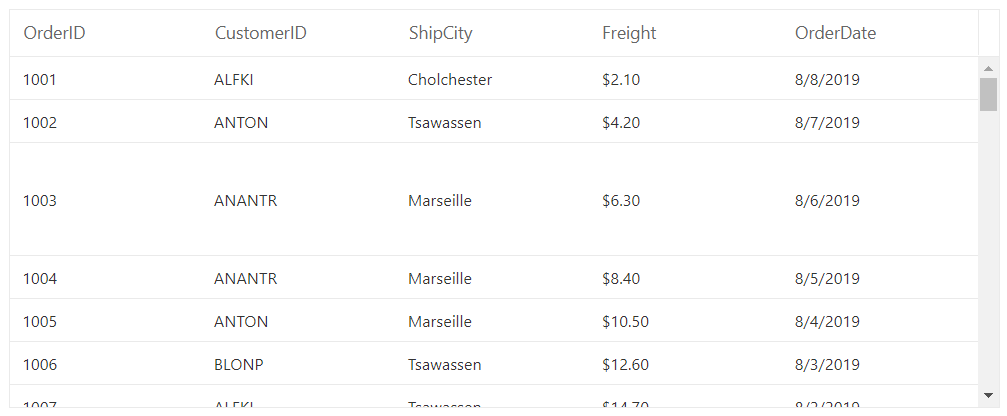# Row Height in Blazor DataGrid Component

16 Aug 20234 minutes to read

You can customize the row height of datagrid rows through the RowHeight property. The RowHeight property is used to change the row height of entire datagrid rows.

In the below example, the `RowHeight` is set as ‘60’.

``````@using Syncfusion.Blazor.Grids

<SfGrid DataSource="@Orders" Height="280" RowHeight="60">
<GridColumns>
<GridColumn Field=@nameof(Order.OrderID) HeaderText="Order ID" Width="110"> </GridColumn>
<GridColumn Field=@nameof(Order.OrderDate) HeaderText="Order Date" Format="d" Width="110" Type="ColumnType.Date"></GridColumn>
</GridColumns>
</SfGrid>

@code {

public List<Order> Orders { get; set; }

protected override void OnInitialized()
{
Orders = Enumerable.Range(1, 75).Select(x => new Order()
{
OrderID = 1000 + x,
CustomerID = (new string[] { "ALFKI", "ANANTR", "ANTON", "BLONP", "BOLID" })[new Random().Next(5)],
ShipCity = (new string[] { "Berlin", "Madrid", "Cholchester", "Marseille", "Tsawassen" })[new Random().Next(5)],
Freight = 2.1 * x,
}).ToList();
}

public class Order
{
public int? OrderID { get; set; }
public string CustomerID { get; set; }
public string ShipCity { get; set; }
public DateTime? OrderDate { get; set; }
public double? Freight { get; set; }
}
}``````## Customize row height for particular row

DataGrid row height for particular row can be customized using the RowDataBound event by setting the height by adding `row-height` class in required row element.

In the below example, the row height for the row with OrderID as ‘1003’ is set as ‘90px’ using the RowDataBound event.

``````@using Syncfusion.Blazor.Grids

<SfGrid DataSource="@Orders" Height="280">
<GridEvents TValue="Order" RowDataBound="RowBound"></GridEvents>
<GridColumns>
<GridColumn Field=@nameof(Order.OrderID) HeaderText="Order ID" Width="110"> </GridColumn>
<GridColumn Field=@nameof(Order.OrderDate) HeaderText="Order Date" Format="d" Width="110" Type="ColumnType.Date"></GridColumn>
</GridColumns>
</SfGrid>

<style>
.row-height {
height: 90px;
}
</style>

@code {

public List<Order> Orders { get; set; }

protected override void OnInitialized()
{
Orders = Enumerable.Range(1, 75).Select(x => new Order()
{
OrderID = 1000 + x,
CustomerID = (new string[] { "ALFKI", "ANANTR", "ANTON", "BLONP", "BOLID" })[new Random().Next(5)],
ShipCity = (new string[] { "Berlin", "Madrid", "Cholchester", "Marseille", "Tsawassen" })[new Random().Next(5)],
Freight = 2.1 * x,
}).ToList();
}

public class Order
{
public int? OrderID { get; set; }
public string CustomerID { get; set; }
public string ShipCity { get; set; }
public DateTime? OrderDate { get; set; }
public double? Freight { get; set; }
}

public void RowBound(RowDataBoundEventArgs<Order> args)
{
if (args.Data.OrderID == 1003)
{